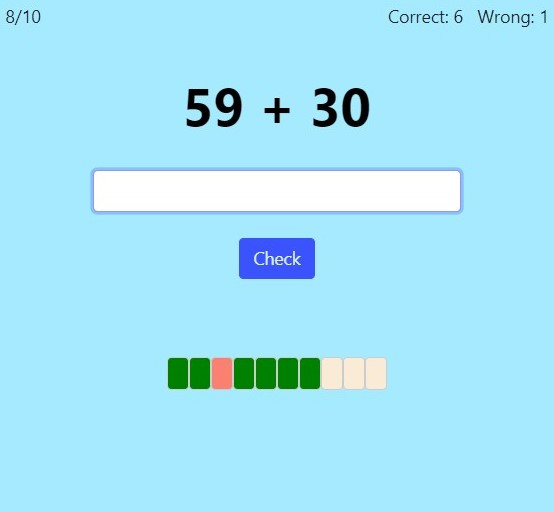MM Practice
×
Multiplication
Division
Place Value
Fractions & Decimals
Measurement
Statistics & Probability
Pre-Algebra
Money
Kindergarten
Geometry

# Two-Digit Addition & Subtraction with Mental MathOnline practice for grades 1-3

Here you can practice adding and subtracting two-digit numbers using mental math (such as 78 + 6, 40 + 15, 54 + 22, 29 + 37, 59 − 4, 100 − 28, etc.).

• First graders should practice — and MASTER — adding a two-digit number and a single-digit number without regrouping (e.g. 24 + 5, 67 + 2), AND adding/subtracting two-digit numbers and multiples of ten (e.g. 45 + 30, 67 − 50).
• For second grade, I suggest practicing adding a two-digit number and a single-digit number with regrouping (e.g. 47 + 8, 77 + 5), and similarly, subtracting a two digit number and a single-digit number with regrouping (e.g. 82 − 4, 64 − 8). Second graders should also add and subtract two-digit numbers without regrouping, and subtract a two-digit number from 100. They can also try adding and subtracting two-digit numbers with regrouping.
• Finally, children in third grade can practice with all the options listed below. 🙂

Screenshot:OR
Practice for a set time: min

Allow my comment to be posted on this site